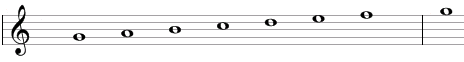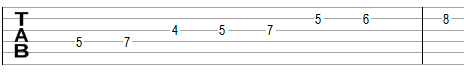# Mixolydian scale

The Mixolydian scale is a primary heptatonic scale that can be built using the following steps between notes: 1, 1, ½, 1, 1, ½, 1.

One example of the scale is G, A, B, C, D, E, F. This example is shown below in traditional notation and in guitar tablature notation.The Mixolydian scale is the fifth mode of standard major scale or the seventh mode of the natural minor scale.

The Mixolydian scale is a "primary" heptatonic scale (heptonia prima) because the intervals between any two adjacent notes are either equal to one semitone or to two semitones and since the two single-semitone intervals are spaced as evenly as possible. Going up from the single-semitone interval between B and C we will find two two-semitone intervals - between C and D and D and E – before we get to the second single semitone interval between E and F. Going down from the single-semitone interval between B and C we will go through three two-semitone intervals – between A and B, G and A, and F and G – before we get to the single-semitone interval between E and F.

## Modes of the Mixolydian scale

The following are modes of the Mixolydian scale.

1. Mixolydian, with steps 1, 1, ½, 1, 1, ½, 1 (G, A, B, C, D, E, F in the example above).
2. Aeolian or natural minor, with steps 1, ½, 1, 1, ½, 1, 1 (A, B, C, D, E, F, G).
3. Locrian, with steps ½, 1, 1, ½, 1, 1, 1 (B, C, D, E, F, G, A).
4. Ionian or major, with steps 1, 1, ½, 1, 1, 1, ½ (C, D, E, F, G, A, B).
5. Dorian, with steps 1, ½, 1, 1, 1, ½, 1 (D, E, F, G, A, B, C).
6. Phrygian, with steps ½, 1, 1, 1, ½, 1, 1 (E, F, G, A, B, C, D).
7. Lydian, with steps 1, 1, 1, ½, 1, 1, ½ (F, G, A, B, C, D, E).

## Three-note chords on the Mixolydian scale

The following are common triads built on the notes of the Mixolydian scale.

• On the root of the scale (on the tonic): major chord (e.g., G composed of G, B, D) or suspended chords (Gsus4 = G, C, D and Gsus2 = G, A, D).
• On the second note (on the supertonic): minor chord (Am = A, C, E) or suspended chords (Asus4 = A, D, E and Asus2 = A, B, E).
• On the third note (on the mediant): diminished chord (Bdim = B, D, F).
• On the fourth note (on the subdominant): major chord (C = C, E, G) or suspended chords (Csus4 = C, F, G and Csus2 = C, D, G).
• On the fifth note (on the dominant): minor chord (Dm = D, F, A) or suspended chords (Dsus4 = D, G, A and Dsus2 = D, E, A).
• On the sixth note (on the submediant): minor chord (Em = E, G, B) or suspended chord (Esus4 = E, A, B).
• On the seventh note (on the leading tone): major chord (F = F, A, C) or suspended chord (Fsus2 = F, G, C).

## Four-note chords on the Mixolydian scale

The following are seventh chords built on the notes of the Mixolydian scale.

• On the first note: dominant seventh chord (e.g., G7 composed of G, B, D, F).
• On the second note: minor seventh chord (Amin7 = A, C, E, G).
• On the third note: half-diminished seventh chord (minor seventh chord with a flat fifth, Bm7b5 = B, D, F, A).
• On the fourth note: major seventh chord (Cmaj7 = C, E, G, B).
• On the fifth note: minor seventh chord (Dmin7 = D, F, A, C).
• On the sixth note: minor seventh chord (Emin7 = E, G, B, D).
• On the seventh note: major seventh chord (Fmaj7 = F, A, C, E).

## Intervals on the Mixolydian scale

The Mixolydian scale is composed of the following intervals.

• A major second, e.g., the interval between G and A is equal to two semitones.
• A major third, e.g., the interval between G and B is equal to four semitones.
• A perfect fourth, e.g., the interval between G and C is equal to five semitones.
• A perfect fifth, e.g., the interval between G and D is equal to seven semitones.
• A major sixth, e.g., the interval between G and E is equal to nine semitones.
• A minor seventh, e.g., the interval between G and F is equal to ten semitones.

Scale, Scale (index)

### Filtered HTML

• Freelinking helps you easily create HTML links. Links take the form of [[indicator:target|Title]]. By default (no indicator): Click to view a local node.